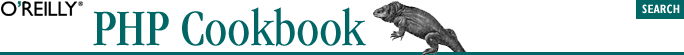home | O'Reilly's CD bookshelfs | FreeBSD | Linux | Cisco | Cisco Exam## 14.9. Storing Encrypted Data in a File or Database

### 14.9.2. Solution

Store the additional information required to decrypt the data (such as algorithm, cipher mode, and initialization vector) along with the encrypted information, but not the key:

```// encrypt data
\$alg  = MCRYPT_BLOWFISH;
\$mode = MCRYPT_MODE_CBC;
\$iv = mcrypt_create_iv(mcrypt_get_iv_size(\$alg,\$mode),MCRYPT_DEV_URANDOM);
\$ciphertext = mcrypt_encrypt(\$alg,\$_REQUEST['key'],\$_REQUEST['data'],\$mode,\$iv);

// save encrypted data
\$dbh->query('INSERT INTO noc_list (algorithm,mode,iv,data) values (?,?,?,?)',
array(\$alg,\$mode,\$iv,\$ciphertext));```

To decrypt, retrieve a key from the user and use it with the saved data:

```\$row = \$dbh->getRow('SELECT * FROM noc_list WHERE id = 27');
\$plaintext = mcrypt_decrypt(\$row->algorithm,\$_REQUEST['key'],\$row->data,
\$row->mode,\$row->iv);```